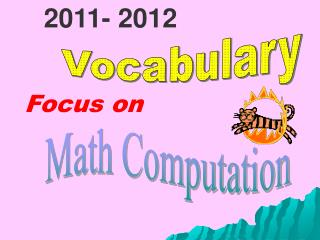DownloadDownload PresentationVocabulary

# Vocabulary

Télécharger la présentation## Vocabulary

- - - - - - - - - - - - - - - - - - - - - - - - - - - E N D - - - - - - - - - - - - - - - - - - - - - - - - - - -
##### Presentation Transcript

1. 2011- 2012 Vocabulary Focus on Math Computation

2. Can you read each word?

3. Can you identify each picture?

4. Can you spell each word correctly?

5. Do you know the definition of each word?

6. whole numbers (0, 1, 3, … )

7. whole numbers whole numbers - the numbers in the set (0,1 ,2, 3,…..)

8. sum

9. sum sum- the answer in addition. Example: 8 + 7 = 15 The sum is 15.

10. difference 9 – 6 = ? 10 – 5 = ?

11. difference difference - the answer in subtraction. Example: 9 – 4 = 5 The difference is 5.

12. product

13. product product: the answer in multiplication. Example: 4 x 8 = 32 The product is 32.

14. quotient

15. quotient quotient: the answer in division. Example: 24 ÷ 3 = 8 The quotient is 8.

16. place value

17. place value place value- the value determined by the position of a digit in a number. Example: In 562, the digit 5 means 5 hundreds, the digit 6 means 6 tens, and the digit 2 means 2 ones.

18. regroup

19. regroup regroup - to rename a number by exchanging base - ten materials of one value for base - ten materials that are equal to it. Example: 12 can be regrouped as 1 ten 2 ones or 12 ones. 253 can be regrouped as 2 hundreds 5 tens 3 ones or 1 hundred 15 tens and 3 ones.

20. array

21. array Array- an arrangement of objects or numbers in rows and columns. Example:

22. numerator

23. numerator numerator – the number above the fraction bar in a fraction. Example: 2/5 (2 is the numerator.)

24. denominator

25. denominator denominator - the number below the fraction bar in a fraction. 2/5 (5 is the denominator.)

26. mixed number

27. mixed number mixed number- a number written as a whole number and a fraction. Example: 2 ¾

28. estimate

29. estimate estimate -a rough guess or calculation about an amount, distance or cost.

30. For more help with these terms and concepts, visit the Online Assessment System at https://georgiaoas.org/servlet/a2l See your child’s teacher for log-in information.